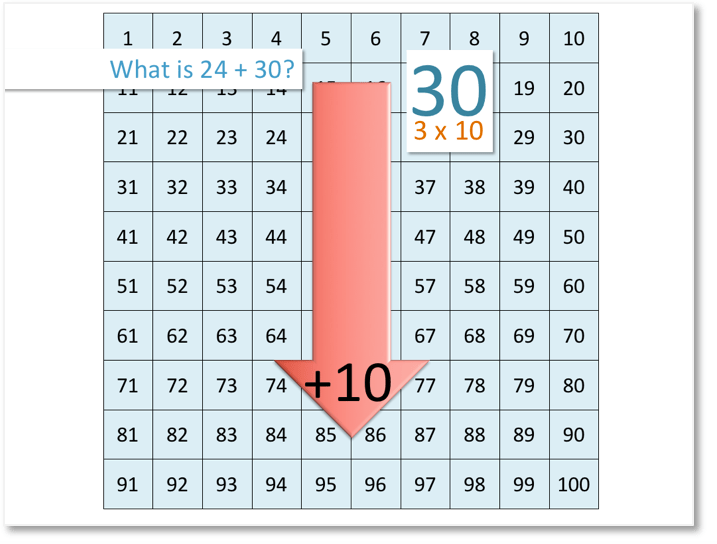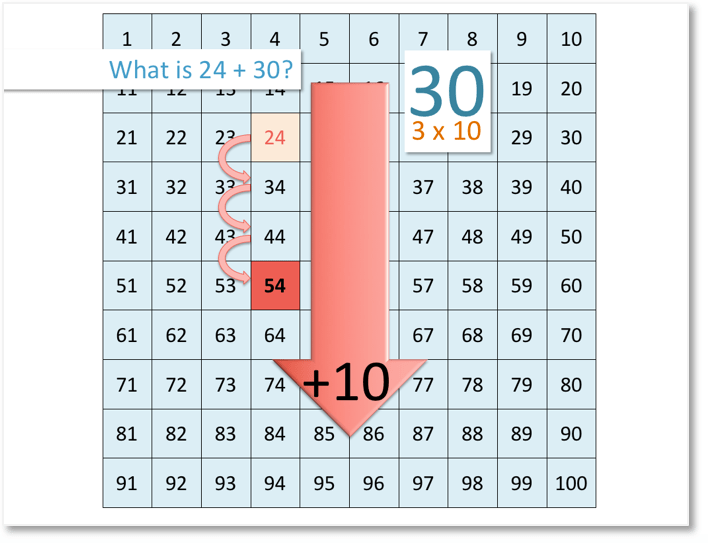# Number Grid: Adding and Subtracting Multiples of Ten

Adding and Subtracting Multiples of Ten: Video Lesson – Maths with Mum Download the Number Grid to 100 below:

Supporting Lessons

## How to Add or Subtract Multiples of 10

When we add 10 to a number using the number grid, we move one place down and when we subtract 10 from a number, we move one place up.

In this lesson, we will look at adding and subtracting multiples of 10 using the number grid.Above are the first nine multiples of 10.

We can see that: 10 is 1 lot of 10

20 is 2 lots of 10

30 is 3 lots of 10

40 is 4 lots of 10

50 is 5 lots of 10

60 is 6 lots of 10

70 is 7 lots of 10

80 is 8 lots of 10

90 is 9 lots of 10

This tells us how many places we move up or down the number grid.

For example, if we are adding 40, we move 4 places down the number grid, or if we are subtracting 50, we move 5 places up the number grid.

Here is an example of addition using the number grid:We are asked: what is 24 + 30?

When we add 10, we move 1 place down the number grid.

30 is 3 lots of 10, so we move 3 places down the number grid.We start at 24 and we move 3 places down the number grid.

We stop at 54. Therefore,

24 + 30 = 54.

Here is an example of subtraction using the number grid:We are asked: what is 82 – 60?

When we subtract 10, we move 1 place up the number grid.

60 is 6 lots of 10, so we move 6 places up the number grid.We start at 82 and we move 6 places up the number grid.

We stop at 22. Therefore,

82 – 60 = 22.